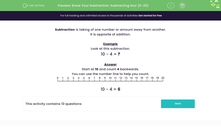# Know Your Subtraction: Subtract 4, 5 or 6 From a Number

In this worksheet, students will practise subtracting 4, 5 or 6 from a given number. (0-20)Key stage:  KS 1

Curriculum topic:   Number: Addition and Subtraction

Curriculum subtopic:   Add and Subtract to 20

Difficulty level:#### Worksheet Overview

Subtraction is the taking of one number or amount away from another.

It is the opposite of addition.

Example

Look at this subtraction.

10 - 4 = ?

Start at 10 and count 4 backwards.10 - 4 = 6

If you use the number line, you start at the number 10 and move 4 places to the left. The number you reach is 6.

Remember that you always move to the left on a number line when you are subtracting.Let's try another one.

Example

16 - 5 = ?

Start at 16 on the number line and count 5 places to the left. What number do you reach? It's 11.

16 - 5 = 11

Does that make sense?Let's move on to some questions now.

### What is EdPlace?

We're your National Curriculum aligned online education content provider helping each child succeed in English, maths and science from year 1 to GCSE. With an EdPlace account you’ll be able to track and measure progress, helping each child achieve their best. We build confidence and attainment by personalising each child’s learning at a level that suits them.

Get started